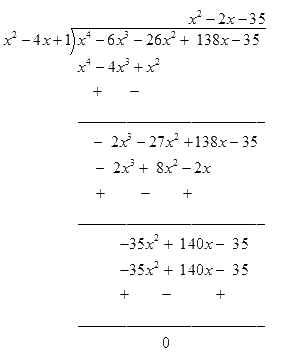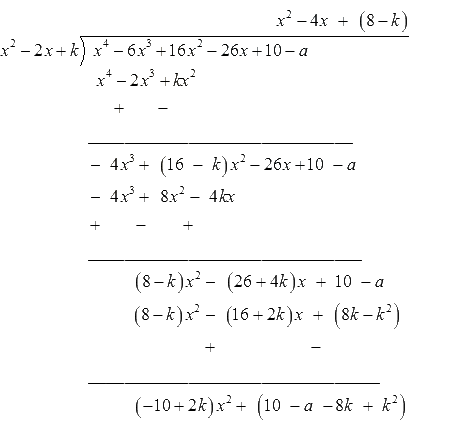# NCERT Solutions For Class 10 Maths Chapter 2 Exercise 2.4

## Chapter 2 Ex.2.4 Question 1

Verify that the numbers given alongside of the cubic polynomials below are their zeroes. Also verify the relationship between the zeroes and the coefficients in each case:

(i) $$\quad 2{x^3} + {x^2} - 5x + 2;\,\,\,\frac{1}{2},\,\,1,\,\, - 2$$

(ii)$$\quad {x^3} - 4{x^2} + 5x - 2;\;,2,\;1,\;1$$

### Solution

What is known?

Cubic polynomials and their zeroes are given

What is unknown?

Verify that the numbers given alongside of the cubic polynomials are their zeroes and verify the relationship between the zeroes and the coefficients in each case.

Reasoning:

This question is very simple, cubic polynomials are already given. Put the values of zeroes in the polynomial, you will get zero because it will satisfy the equation of the given polynomial. Now compare the polynomial with $$a{x^3} + b{x^2} + cx + d,$$ you will get the values of the coefficients of $$x$$ i.e. $$a, \,b,\, c$$ and $$d.$$

Steps:

$$p\left( x \right) = 2{x^3} + {x^2}-5x + 2$$

Given numbers are $$= \frac{1}{2},\;1,\; - 2$$

Put $$x= \frac{1}{2}$$ in $$p\left( x \right) = 2{x^3} + {x^2}-5x + 2$$

\begin{align}p\left( {\frac{1}{2}} \right) & \!\!=\!\! 2 \! {\left( {\frac{1}{2}} \right)^3} \!\!+\!\! {\left( {\frac{1}{2}} \right)^2}\! \!- 5 \! \left( {\frac{1}{2}} \right) \!\!+\! \! 2\\p\left( {\frac{1}{2}} \right) &\!\!= \!\!2\left( {\frac{1}{8}} \right) \!\!+ \!\! \left( {\frac{1}{4}} \right) - 5\left( {\frac{1}{2}} \right) \!+\!\! 2\\p\left( {\frac{1}{2}} \right) &= \frac{1}{4} + \left( {\frac{1}{4}} \right) - \frac{5}{2} + 2\\p\left( {\frac{1}{2}} \right)& = \frac{{1 + 1 - 10 + 8}}{4}\\p\left( {\frac{1}{2}} \right) &= 0\end{align}

Put $$x= 1$$ in $$p\left( x \right) = 2{x^3} + {x^2}-5x + 2$$

\begin{align}p\left( 1 \right)&= 2{\left( 1 \right)^3} + {\left( 1 \right)^2} - 5\left( 1 \right) + 2\\p\left( {\frac{1}{2}} \right) &= 2 + 1 - 5 + 2\\p\left( 1 \right) &= 3 - 5 + 2\\p\left( 1 \right) &= 0\end{align}

Put $$x= - 2$$ in $$p\left( x \right) = 2{x^3} + {x^2}-5x + 2$$

\begin{align}{p}\left( { - 2} \right)&= \!\!2\left( - 2 \right)^3 + \left( - 2 \right)^2 - 5( - 2) \!\!+ \!\!2\\{{p}\left(- 2 \right)} &= {16 - 8 + 4 + 10 + 2}\\{{p}\left( { - 2} \right)}& ={ - 16 + 4 + 10 + 2}\\{{p}\left( { - 2} \right)}&={ - 16 + 16}\\{p\left( { - 2} \right)} &= 0\end{align}

Therefore, \begin{align}\frac{1}{2},\;1,\; - 2 \end{align} are the zeroes of the polynomial.

Now let  \begin{align}\alpha = \frac{1}{2},\,\,\beta = 1 \end{align} and \begin{align}\gamma = 2.\end{align}

\begin{align}\alpha + \beta + \gamma &= \frac{1}{2} + 1 + \left( { - 2} \right)\\ &= \frac{{ - 1}}{2}\\&= \frac{{ -{ \text{coeficient of }}{x^2}}}{{{\text{coeficient of }}{x^3}}}\end{align}

\begin{align} & \alpha \beta + \beta \gamma + \gamma \alpha \\ \\ &=\! \frac{1}{2}\! \!\times\!\! 1 \!+ \!1\!\! \times\! \left( {\!\! - \!2} \right) \!+\! \left( {\!\! -\! 2} \right) \!\!\times\! \!\frac{1}{2}\\ &= \frac{{ - 5}}{2}\\& = \frac{{ - {\text{coeficient of }}x}}{{{\text{coeficient of }}{x^3}}}\end{align}

\begin{align}\alpha .\beta .\gamma &= \frac{1}{2} \times 1 \times \left( { - 2} \right)\\&= \frac{{ - 2}}{2}\\ &= \frac{{ - {\text{ constant term }}}}{{{\text{ coeficient of }}{x^3}}}\end{align}

Hence, the relation between zeroes and coefficient is verified.

(ii)$$\quad {x^3} - 4{x^2} + 5x - 2;\;,2,\;1,\;1$$

Given numbers are \begin{align} = 2,1,1\end{align}

Put $$x = 2$$ in $${x^3}-4{x^2} + 5x-2$$

\begin{align}{p\left( 2 \right)} &= {{{\left( 2 \right)}^3} - 4{{\left( 2 \right)}^2} + 5\left( 2 \right) - 2}\\{p\left( 2 \right)}& = {8 - 16 + 10 - 2}\\{p\left( 2 \right)} & ={ 2 - 2}\\{p\left( 2 \right)} &= 0\end{align}

Put $$x = 1$$ in $${x^3}-4{x^2} + 5x-2$$

\begin{align}p\left( 1 \right) &= {{\left( 1 \right)}^3} - 4{{\left( 1 \right)}^2} + 5\left( 1 \right) - 2\\p\left( 1 \right)& = 1 - 4 + 5 - 2\\p \left( 1 \right)&= - 3 + 3\\p \left( 1 \right) &= 0\end{align}

Therefore, $$2,\,1$$ and $$1$$ are the zeroes of the polynomial.

Now let  $$\alpha = 2,\;\beta = 1$$ and $$\gamma = 1.$$

\begin{align}\alpha + \beta + \gamma &= 2 + 1 + 1\\& = \frac{{ - \left( { - 1} \right)}}{1}\\&= \frac{{ - \,{\text{coeficient of }}{x^2}}}{{{\text{coeficient of }}{x^3}}}\end{align}

\begin{align}\alpha \beta + \beta \gamma + \gamma \alpha&= 2\! \times \!1\! + \!1\! \times \!1 \!+\! 1\! \times\! 2 \! \\& = \!5\\&= \frac{5}{1}\\&= \frac{{ - \,{\text{coeficient of }}x}}{{{\text{coeficient of }}{x^3}}}\end{align}

\begin{align}\alpha \,.\,\,\beta \,.\,\,\gamma &= \;2\; \times \;1\; \times \;1\;\; = \;2\\ &= \frac{{ - \left( { - 2} \right)}}{1}\\& = \frac{ -\,\text{constant term}}{\text{coeficient of }{x^3}}\end{align}

Hence, the relation between zeroes and coefficient is verified.

## Chapter 2 Ex.2.4 Question 2

Find a cubic polynomial with the sum, sum of the product of its zeroes taken two at a time, and the product of its zeroes as $$2, –7, –14$$ respectively.

### Solution

What is known?

Zeroes of a Cubic polynomials are $$2, –7, –14$$ respectively.

What is unknown?

A cubic polynomial with the sum, sum of the product of its zeroes taken two at a time.

Reasoning:

To solve this question, follow the steps below-

We know that the general equation of the polynomial is $$a{x^3} + {{ }}b{x^2} + cx{{ }} + {{ }}d$$ and the zeroes are $$\alpha ,\;\beta$$ and $$\gamma .$$

Now we have to find the sum, sum of the product of its zeroes taken two at a time, and the product of its zeroes. For this we know that,

\begin{align}{\alpha + \beta + \gamma}& = {\frac{{ - b}}{a}}\\{\alpha \beta + \beta \gamma + \gamma \alpha}& = {\frac{c}{a}}\\{\alpha \beta \gamma}& = \frac{{ - d}}{a}\end{align}

Put the values of the known coefficients, you will get the value of unknown coefficient.

Now put the values of coefficients in the general equation of the cubic polynomial $$a{{x}^{3}}+{ }b{{x}^{2}}+cx+d.~$$

Steps:

Let the polynomial be

$$a{x^3} + {{ }}b{x^2} + cx + d$$ and the zeroes are $$\alpha,\;\beta$$  and $$\gamma.$$

We know that

\begin{align}\alpha + \beta + \gamma &= \frac{2}{1}\\ &= \frac{{ - b}}{a}\\\\\alpha \beta + \beta \gamma + \gamma \alpha &= \frac{{ - 7}}{1}\\ &= \frac{c}{a}\\\\\alpha \beta \gamma &= \frac{{ - 14}}{1}\\& = \frac{{ - d}}{a}\end{align}

if $$a = 1$$ then $$b = - 2, \;c = - 7$$ and $$d = 14$$

Hence, the polynomial is $${x^3} - 2{x^2} - 7x + 14$$

## Chapter 2 Ex.2.4 Question 3

If the zeroes of the polynomial

$$x^{3}-3 x^{2}+x+1$$ are $$a-b, \;a,\;a+b,$$

find $$a$$ and $$b$$.

### Solution

What is known?

Zeroes of the polynomial $$x^{3}-3 x^{2}+x+1$$ are $$a- b, \; a,\; a+b$$

What is unknown?

$$a$$ and $$b$$.

Reasoning:

First compare the given polynomial with the general equation of the cubic polynomial $$p{x^3} + q{x^2} + rx + t$$ and you will get the values of $$p, \;q,\; r$$ and $$t.$$

Now put this value in the equation of sum of zeroes and product of zeroes, you will get the value of $$a$$ and $$b$$.

Steps:

$p\left( x \right) = {x^3} - 3{x^2} + x{{ }} + 1$

Zeroes are $$a - b,{{ }}a,\;a + b.$$

On comparing the given polynomial with $$p{x^3} + q{x^2} + rx + t$$

we get,

$$p = 1, \;q = - 3,\;r = 1$$ and $$t = 1$$

Sum of zeroes

\begin{align}&= a - b + a + a + b\\& = \frac{{ - {\text{coeficient of }}{x^2}}}{{{{ }}{\text{coeficient of }}{x^3}}} = \frac{{ - q}}{p}\\{\frac{{ - q}}{p}}&={ 3{{a}}}\\{\frac{{ - ( - 3)}}{1}} &= {3{{a}}}\\a{{ }}& = {{ }}1\end{align}

Since the value of $$a$$ is found to be $$1,$$ the zeroes are $$1 - b,\;1,\;1 + b$$

Multiplication of zeroes

\begin{align}&= 1\left( {1 - b} \right)\left( {1 + b} \right)\\& = \frac{{ - {\text{constant term}}}}{\text{coeficient of }{x^3}}\\&= \frac{{ - t}}{p}\\{\frac{{ - t}}{p}} &= {1-{{ }}{b^2}}\\\frac{{ - 1}}{1} &= {1-{{ }}{b^2}}\\1 - {b^2}& = 1\\1 + 1 &= {b^2}\\{b^2} &= 2\end{align}

$$b = \pm \sqrt 2 .$$ Hence, $$a = 1, \;b = \sqrt 2$$ or $$- \sqrt 2$$

## Chapter 2 Ex.2.4 Question 4

If two zeroes of the polynomial

$${x^4}-6{x^3}-26{x^2} + 138x-35$$ are $$2 \pm \sqrt 3$$

find other zeroes.

### Solution

What is known?

Two zeroes of the polynomial

$$x^{4}-6 x^{3}-26 x^{2}+138 x-35$$ are

$$2 \pm \sqrt 3 .$$

What is unknown?

Other zeroes of the given polynomial.

Reasoning:

Given polynomial and the zeroes of the polynomial are

$$x^{4}-6 x^{3}-26 x^{2}+138 x-35$$ and

$$2 \pm \sqrt 3 .$$

By using the zeroes of a polynomial, you can find out the factor of the polynomial.

Now divide the polynomial with the factor, you will get the quotient and remainder of the polynomial.

Put this value in the division algorithm and you will get the other zeroes by simplifying its factors.

Steps:

$P\left( x \right) \!= \!{x^4} \!- \! 6{x^3} \!- \!26{x^2} \!+\! 138x \! - \! 35$

Zeroes of the polynomial are $$= 2 \pm \sqrt 3 .$$

Therefore,

\begin{align}&\left( {x - 2 + \sqrt 3 } \right)\left( {x-2 - \sqrt 3 } \right) \\&= {{ }}{x^2} + 4-4x - 3\\&= {{ }}{x^2}-{{ }}4x{{ }} + {{ }}1\end{align}

is a factor of the given polynomial.

To find out the other polynomial, we have to find the quotient by dividing

$${x^4}-6{x^3}-26{x^2} + 138x-35$$ by

$${x^2}-4x + 1$$Clearly, by division algorithm,

\begin{align}&{x^4}-6{x^3}-26{x^2} + 138x-35\\&=\left( {{x^2}-4x+ 1} \right)\left( {{x^2} - 2x- 35} \right)\end{align}

It can be observed that $${x^2} - 2x - 35$$ is a factor of the given polynomial and

\begin{align}{x^2} - 2x - 35 &= {x^2} - 7x + 2x - 35\\ &= \left( {x - 7} \right)\left( {x - 5} \right)\end{align}

Therefore, the value of the polynomial is also zero when $$x-7 = 0$$ or $$x + 5 = 0$$

Or $$x=7$$ and $$x\!=\!-5$$

Hence, $$7$$ and $$\!-\!5$$ are also zeroes of this polynomial.

## Chapter 2 Ex.2.4 Question 5

If the polynomial $${x^4}-{{ }}6{x^3} + {{ }}16{x^2}-{{ }}25x + {{ }}10$$ is divided by another polynomial $${x^2}-{{ }}2x + k,$$ the remainder comes out to be $$x + a,$$ find $$k$$ and $$a.$$

### Solution

What is known?

If the polynomial $${x^4}-6{x^3} + {{ }}16{x^2}-25x + {{ }}10$$ is divided by another polynomial $${x^2}-2x + k,$$ the remainder comes out to be $$x+a.$$

What is unknown?

Value of $$k$$ and $$a.$$

Reasoning:

This question is straight forward, use division algorithm and put the known values of dividend, divisor and remainder in the division algorithm and get the Quotient. Now you will get the remainder, but we have already subtracted remainder that means ideally the remainder should be equal to zero. Now, on equating remainder with zero, you will get the value of $$k$$ and $$a.$$

Steps:

By Division algorithm,

Dividend $$=$$ Divisior $$\times$$ Quotient  $$+$$  Remainder

\begin{align}\begin{bmatrix}{x^4}\! -\! 6{x^3} \!+ \!16{x^2} \\\! -\! 25x + 10\end{bmatrix} &\!=\! \!\begin{bmatrix}\left( {{x^2} \!- \!2x \!+\! k} \right) \\ \! \times \! Q \!+\! \left( {x \!+\! {{a}}} \right)\end{bmatrix}\\ \begin{bmatrix}{x^4} \!-\! 6{x^3} \!+ \!16{x^2} \!- \!\\26x\! +\! 10 \!-\! a \end{bmatrix}&\!=\! \!{\left( {{x^2}\! - \!2x \!+ \!k} \right) \!\times\! Q}\\{\frac{\begin{bmatrix}{x^4} - 6{x^3} - 26x \\+ 10 - a\end{bmatrix}}{{\left( {{x^2} - 2x + k} \right)}}} &= Q\end{align}On comparing

\begin{align} \left( -10+2k \right)&=0 \\ 2k&=10 \\k & = 5 \\\end{align}

\begin{align}10 - a - 8k + {k^2}& = 0\\10 - a - 8(5) + {{(5)}^2} &= 0\\10 - a - 40 + 25 &= 0\\- 30 + 25 - a &= 0\\- 5 - a &= 0\\a &= - 5\end{align}

Hence \begin{align} k = 5, \;a = - 5 \end{align}

Download SOLVED Practice Questions of NCERT Solutions For Class 10 Maths Chapter 2 Exercise 2.4 for FREE
Ncert Class 10 Exercise 2.4
Ncert Solutions For Class 10 Maths Chapter 2 Exercise 2.4
Instant doubt clearing with Cuemath Advanced Math Program